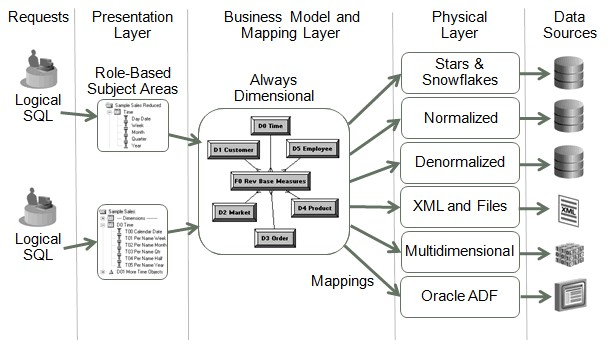# OBIEE - How to produce and show a total section on each line (Running total)?

This can be achieved by aggregation using the BY aggregate clause.

You can calculate a specified level of aggregation using BY within the aggregate function itself.

## How-to / Example

Add a duplicate copy of the measure column to your request and edit the column formula for this and set it to sum( BY ).

This will show the sum of the total sum of measure for the group that the current row is associated to.

To illustrate this within a query for e.g.

````select year, product, revenue, sum(revenue by year) as year_revenue from softdrinks`
```

So using the above query example, within a request you would add 3 columns year, product and revenue from softdrinks table. And then add another copy of revenue column again. Modify the column name for this duplicate column to year_revenue. And set it's column formula to sum(revenue by year).

The results for reference would be as follows:

``````Year Product Revenue Year_Revenue
2001 P01      10           30 (i.e. 10+20)
2001 P02      20           30 (i.e. 10+20)
2002 P01      30           70 (i.e. 30+40)
2002 P03      40           70 (i.e. 30+40)
2004 P02      50           50 (i.e 50)
```
```

Recommended PagesOBIEE - Logical Sql

The logical SQL is the SQL that understand BI Server. It's a full Ansi 92 SQL (including subqueries and derived tables), plus special functions (SQL extensions) like AGO, TODATE, EVALUATE, and others....